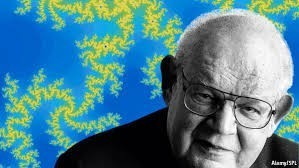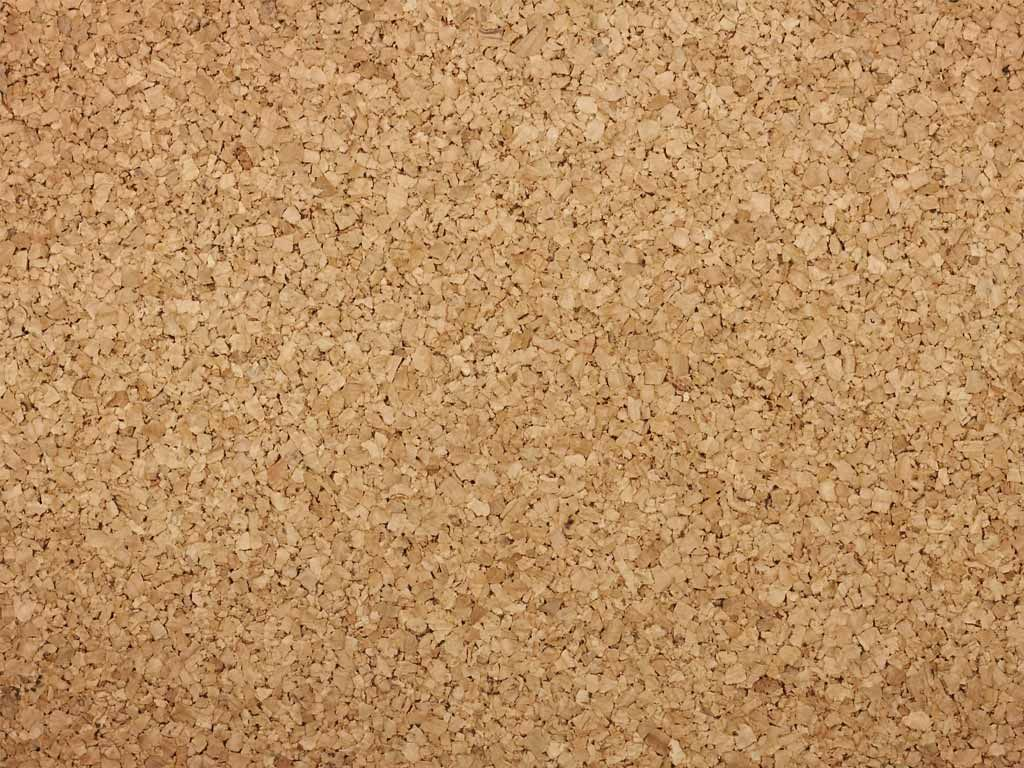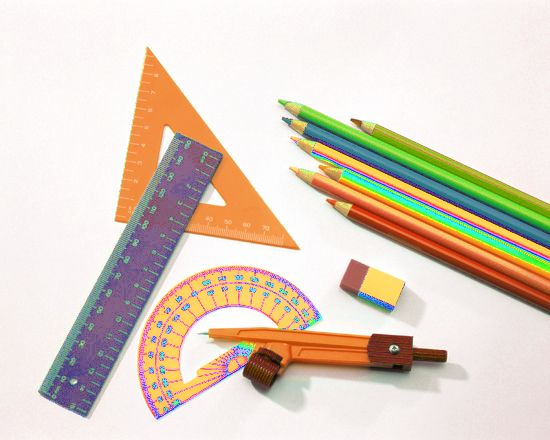Fractal Geometry

The mathematician pictured is Benoit Mandelbrot, the "father " of FRACTAL Geometry.

Below are helpful websites for Geometry Class.Simplifying Radicals Go here for Review
Ceva's Theorem- Really cool! And a proof too!   2nd Proof- link
Circles : Coordinate Geometry & EuclideanMath Worksheets To Go-Radicals-this includes You Tube
Volumes and Surface AreaMath IS FUN link congruent trianglesTheorems, Postulates other
A MUST KNOW list​
Medians, Altitudes, Angle Bisectors
Ultimate Geometry Review sheet-from Bronx Science- it has lots of examples and details.
Resources